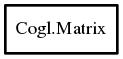# Matrix

## Object Hierarchy:## Description:

public struct Matrix

A CoglMatrix holds a 4x4 transform matrix.

This is a single precision, column-major matrix which means it is compatible with what OpenGL expects.

A CoglMatrix can represent transforms such as, rotations, scaling, translation, sheering, and linear projections. You can combine these transforms by multiplying multiple matrices in the order you want them applied.

The transformation of a vertex (x, y, z, w) by a CoglMatrix is given by:

`  x_new = xx * x + xy * y + xz * z + xw * w  y_new = yx * x + yy * y + yz * z + yw * w  z_new = zx * x + zy * y + zz * z + zw * w  w_new = wx * x + wy * y + wz * z + ww * w`

Where w is normally 1

<note>You must consider the members of the CoglMatrix structure read only, and all matrix modifications must be done via the cogl_matrix API. This allows Cogl to annotate the matrices internally. Violation of this will give undefined results. If you need to initialize a matrix with a constant other than the identity matrix you can use init_from_array.</note>

Namespace: Cogl
Package: doc